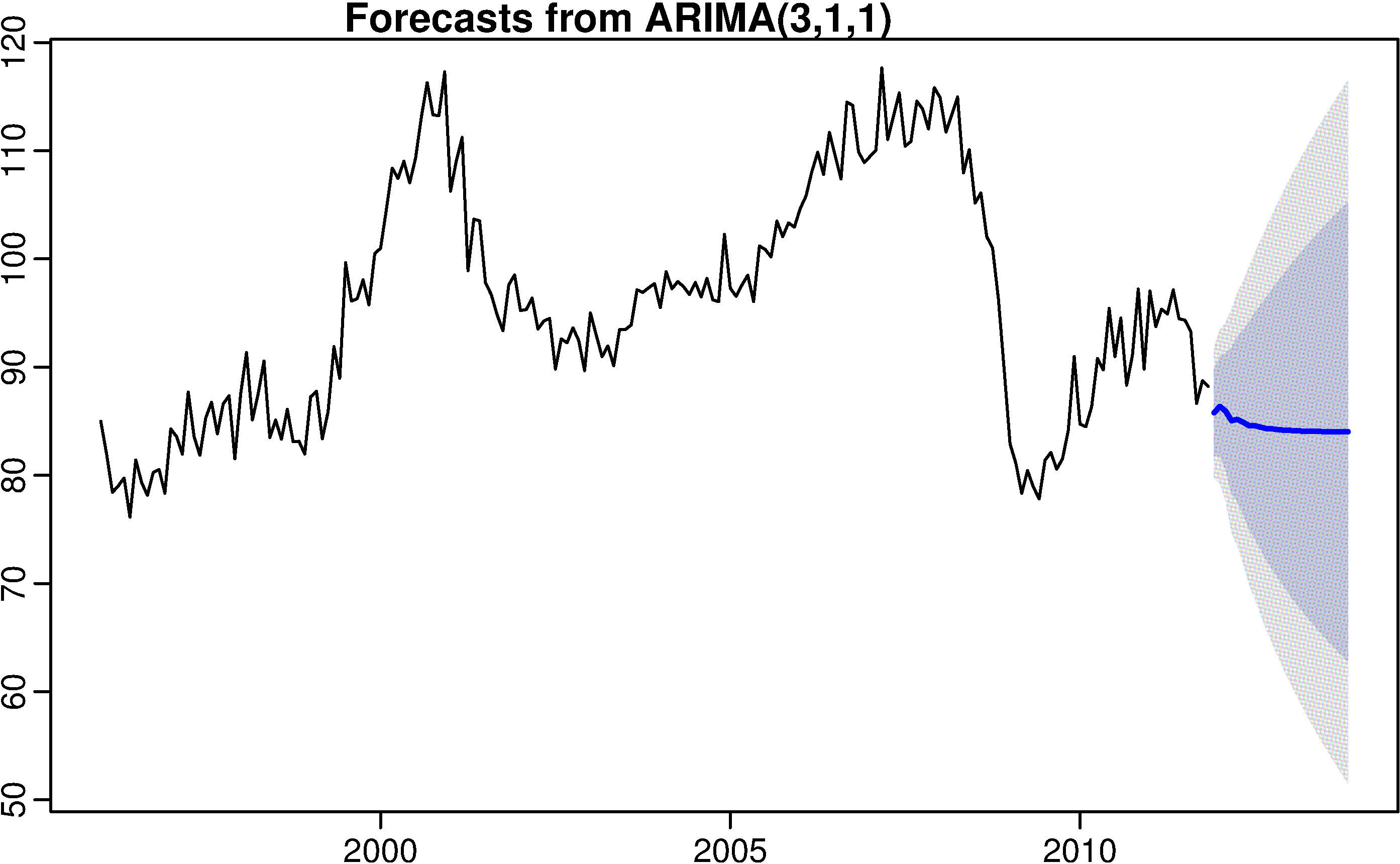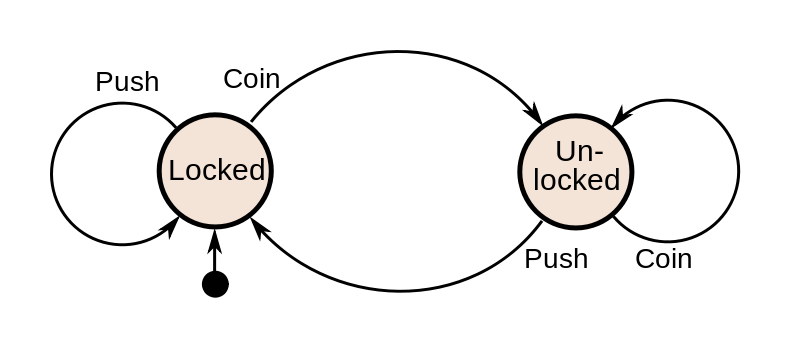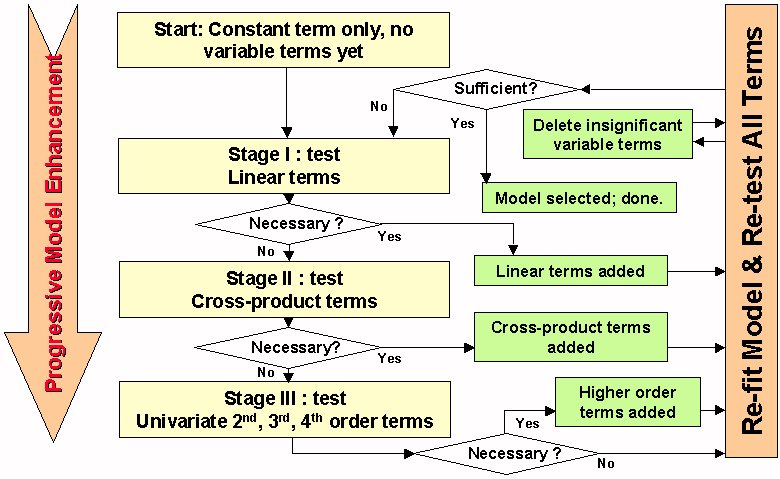# Market forecasting

• Self-optimizing ARIMA expert
• Finite Impulse Response Neural Network
• Finite State Markov Automation
• Stepwise Best Regression
• Square Root Regression
• Square Regression
• Logistic RegressionSelf-optimizing ARIMA expert

• Full ARIMA(p,d,q) implementation
• Unlimited order of mixed modeling
• Conditional error estimates
• Chi-square statistics on residuals
• Expert inference for optimal parameters
• Prediction on multiple future horizons

FIR Neural Network

• Finite-Impulse-Response (FIR)
• Optimal selection of filter parameters
• Temporal back-propagation algorithmFinite State Markov Automation

• Market data flow exploration
• Dynamically construct Markov models
• Building state transition graph
• Predict future market statesStepwise Regression Algorithm

• Enter and remove predictors, in a stepwise manner, until there is no justifiable reason to enter or remove more.
• At each step, enter or remove a predictor based on partial F-tests.
• Stop when no more predictors can be justifiably entered or removed from the stepwise model.

History Prophet

• Dummy predictor for strategy testing
• Predicts every point with its future value
• Imitates a “prophet” knowing the future
• Delivers 100% of profitable trades
• Explicitly uses forward info
• Not suitable for practical trading
• Analog of “Maximum Profit System”

Extensible algorithmic API

• Modular algorithmic server
• Extendable calculation engine
• Real-time C++ core framework
• Open standard development API
• Universal DLL interface
• Compatibility with development tools
• Multiple sample models